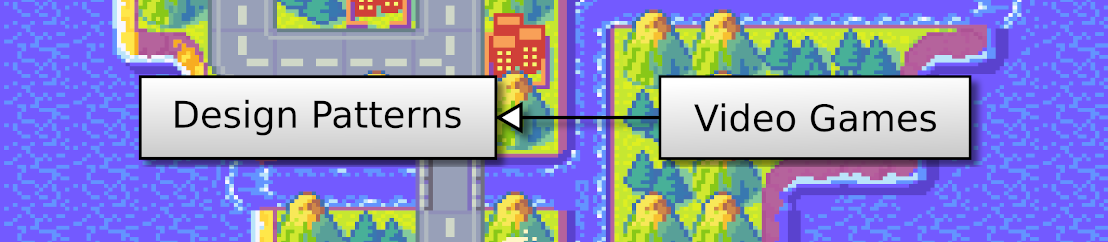## OpenGL 2D Facade (27): Earthquake effect

In this post, I show how to get an earthquake effect (or screen shaking). As before, OpenGL does most of the job, and there is nearly no overhead!

This post is part of the OpenGL 2D Facade series

## Objective

I propose different effects; you can see them here:

We use the same vertex shader as before, where we add a shift to all vertices using a uniform variable:

``````#version 330
layout (location=0) in vec4 vertex;
layout (location=1) in vec2 inputUV;
out vec2 outputUV;
uniform vec4 translation;
uniform vec2 uvShift;
void main() {
gl_Position = vertex + translation;
outputUV = inputUV + uvShift;
}``````

The final vertex position of each quad/tile is the one of the vertex plus a translation:

``    gl_Position = vertex + translation;``

We set the value of the `translation` uniform variable with the Opengl `glUniform4f()` function:

``````translationShaderVar = glGetUniformLocation(shaderProgramId, "translation")

We only consider 2D translations, so there is only an `x` and `y` value. The `shaderProgramId` variable contains the id of our shader.

## Layer groups

We still store in two attributes, `translationX` and `translationY`, the view shift of a layer group. We use them, for instance, to center the world around the player.

We could also change these values to add an effect, but it would be more complex to handle. We would mix a translation that corresponds to some constraints (like centering around the player) with a purely visual effect. It is easier to split these shifts into different attributes and sum them before transferring them to the shader. Each one has its rules, and we can handle them separately.

Consequently, we add a new `shiftEffectX`, `shiftEffectY` attribute pair in each layer group. Then, during the drawing, we add the shifts (see the `render()` method of `OpenGLGUIFacade` class):

``````translationShaderVar = glGetUniformLocation(self.__shaderProgramId, "translation")
for layerGroup in self.__layerGroups:
if layerGroup is None:
continue
x = layerGroup.translationX + layerGroup.shiftEffectX
y = layerGroup.translationY + layerGroup.shiftEffectY
for layer in layerGroup:
if layer is None:
continue
layer.draw()``````

Finally, when we want to add a shift for effects, we use a new `setShiftEffect(x, y)` method in the `LayerGroup` class.

## Earthquake effects

As we can see in the video above, there are different ways to shake the screen. In each case, we compute a shift for each frame considering a function. Note that we only compute horizontal shifts, but you can easily extend to vertical ones if you wish.

### Random

``````f = random.uniform(-1, 1)
x = f * 32
self.__layerGroup.setShiftEffect(x, 0)``````

We ask for a random value between -1 and 1 (line 1), multiply it by 32 to get shifts up to 32 pixels (line 2), and send that value to the layer group.

### Sinus

To get something more regular, we can use the sinus function:

``````f = math.sin((time - start) / 25)
x = f * 32
self.__layerGroup.setShiftEffect(x, 0)``````

The `time` variable contains the current time in milliseconds, and `start` the time when the effect started. As a result, `(time - start)` is the number of milliseconds since the effect started. We divide it by 25 and compute the sinus of the result (line 1). You can change this value to get a different shake rate. The sinus function always returns values between -1 and 1, and `sin(0)` equals 0. Consequently, `f` starts with a zero value, increases to 1, decreases to -1, comes back to 1, and so on.

We can get a more exciting effect with some fading:

``````f = ...
m = 1.0 - (time - start) / (end - start)
x = f * m * 32
self.__layerGroup.setShiftEffect(x, 0)``````

The `m` variable goes from 1 to 0. It equals 1 when the effect starts (the current time equals `start`), and equals 0 when the effect ends (the current time equals `end`).

## Implementation

We implement these shift computations in the `RegionRenderer` class in the `render` package.

We add new attributes to trigger and manage this effect:

``````self.__earthquakeType = ""
self.__earthquakeStart = 0.0
self.__earthquakeEnd = 0.0``````

The `earthquakeType` attribute defines the effect function: "random" or "sinus" (empty string means no effect). The `earthquakeFade` attributes can define a fade function: "linear" or nothing. The two last attributes define the beginning and the end of the effect.

We can set these values with a new `startEarthquake()` method in the `RegionRenderer` class. You can see examples of calls in the `PlayGameMode` class in the `mode` package, where we trigger earthquakes when the player presses a key.

The `updateAnimations()` method of the `RegionRenderer` class handles the computation of the shift:

``````def updateAnimations(self, time: float, epochFrame: float):
for renderer in self.__renderers:
renderer.updateAnimations(time, epochFrame)

if self.__earthquakeType == "":
self.__layerGroup.setShiftEffect(0, 0)
return

if time >= self.__earthquakeEnd:
self.__earthquakeType = ""
self.__layerGroup.setShiftEffect(0, 0)
return

f = 0
if self.__earthquakeType == "random":
f = random.uniform(-1, 1)
elif self.__earthquakeType == "sinus":
f = math.sin((time - self.__earthquakeStart) / 25)
m = 1.0
m = 1.0 - (time - self.__earthquakeStart) / (self.__earthquakeEnd - self.__earthquakeStart)
x = f * m * 32
self.__layerGroup.setShiftEffect(x, 0)``````

Lines 2-3 are the previous animation handling.

Lines 5-7 leave if there is no effect to run.

Lines 9-12 leave if the effect is over.

Lines 14-23 compute the shift.